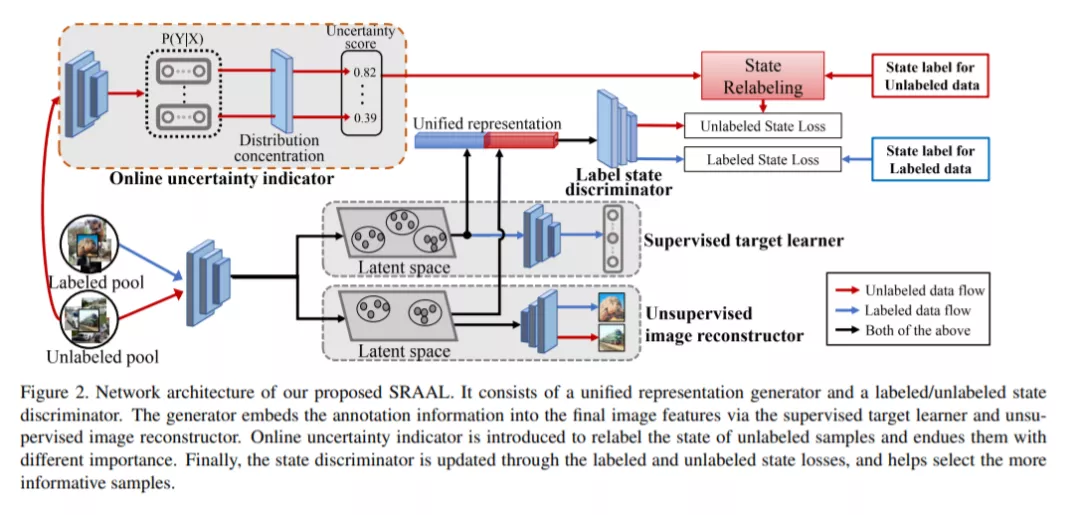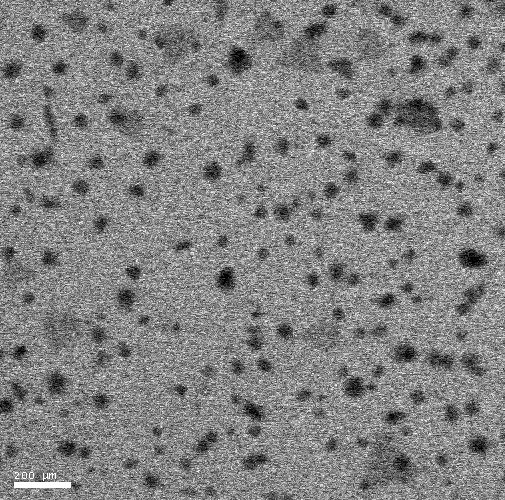### VIP内容0170

### 热门内容000

### 最新内容000

### 最新论文Selecting input variables or design points for statistical models has been of great interest in sequential design and active learning. Motivated by two scientific examples, this paper present a strategy of selecting the design points for a regression model when the underlying regression function is discontinuous. The first example is compressive material imaging with the purpose of accelerating the imaging speed, and the second example is a sequential design for learning a phase diagram in chemistry. In both examples, the underlying regression functions have discontinuities, so many of the existing design optimization approaches cannot be applied for the two examples, because they mostly assume a continuous regression function. There are a few studies for estimating a discontinuous regression function from its noisy observations, but all noisy observations are typically provided in advance in these studies. In this paper, we develop a design strategy of selecting the design points for regression analysis with discontinuities. We first review the existing approaches relevant to design optimization and active learning for regression analysis and discuss their limitations in handling a discontinuous regression function. We then present our novel design strategy for a regression analysis with discontinuities: some statistical properties with a fixed design will be presented first, and then these properties will be used to propose a new criterion of selecting the design points for the regression analysis. Sequential design with the new criterion will be presented with comprehensive simulated examples, and its application to the two motivating examples will be presented.

•000下载预览
Top